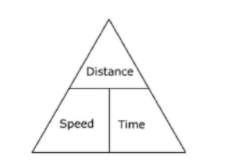A train leaves Delhi at 10 am. and reaches Jaipur at 4 p.m. on the same day. Another trainleaves Jaipur at 12 p.m. and reaches Delhi at 5 p.m. on the same day. What is the time of day(approximately) when the two trains will meet ?(A) 1.42 pm(B) 1.27 pm(C) 04 pm(D) 1.49 pmVerified
146.4k+ views
Hint: Distance- Length of line joining two given points is called distance between them.
Unit of distance - metre(m), kilometer(km), centimeters (cm) etc.
Speed- Distance covered per unit time is called speed or we can say that ratio of distance and
Time is known as speed.
Unit of speed - m/s (meter/second) , km/s(kilometer per second) , km/hr ( kilometer per hour)
etc.
Formulas for speed, distance and time are –
Speed $= \dfrac{{Dis\tan ce}}{{Time}}$
Distance $=$ Speed $\times$ Time
Time $= \dfrac{{Dis\tan ce}}{{Speed}}$
We can easily learn the above formulas with the help of this triangle.The positions of the words in the triangle will help us to learn these formulas.

To find the speed, distance is over time in the triangle, so speed is distance divided by time.

To find distance, speed is beside time, so distance is speed multiplied by time.
Average speed-:
If an object covers different distances with different speeds, then average speed of that object
is an indication of the average rate at which it covers that particular distance.
Or
Average Speed $=$ (Total distance covered throughout the journey) $/$ (Total time taken for the journey)
Average speed $= \left( {D_1 + D_2 + D_3 + \ldots } \right)/\left( {t_1 + t_2 + t_3 + \ldots } \right)$

Complete step by step solution: Time of leaving Delhi by train 1 $=$ 10 AM
Time on which train 1 reached Jaipur $=$ 4 PM
Time of leaving Jaipur by train 2 $=$ 12 PM
Time on which train 2 reached Delhi $=$ 5 PM
We need to find the time when both trains meet.
For train 1
Total time taken to reach Jaipur $=$ 4 PM – 10 AM $=$ 6 hours
Let the distance between 2 stations be x km
Then, speed of train 1 $= \dfrac{{Distance}}{{Time}}$
${S_1} = \dfrac{x}{6}km/hr$ …..(1)
For train 2
Total time taken to reach Delhi $=$ 5 PM – 12 PM $=$ 5 hours
Since, distance $=$ x km
So, speed of train 2 $= \dfrac{{Dis\tan ce}}{{Time}}$
${S_2} = \dfrac{x}{5}km/hr$ ….. (2)
Let both trains meet at time $'t'$, distance covered by train ${T_1}$ after time $'t'$
${D_1} =$ speed $\times$ time
${D_1} = {S_1} \times t$
${D_1} = \dfrac{x}{6}t$ …..(3)
Since train 2 leaves the station 2 hours later then train 1.
So distance covered by train 2,
${D_2} =$ speed $\times$ time
$= {S_2} \times (t - 2)$
${D_2} = \dfrac{x}{5}(t - 2)$ …..(4)
We know that the total distance between 2 stations is x.
And also $x = {D_1} + {D_2}$
So, $x = \dfrac{{xt}}{6} + \dfrac{x}{5}(t - 2)$
$t = \dfrac{t}{6} + \dfrac{t}{5} - \dfrac{2}{5}$
$30 = 11t - 12$
$11t = 42$
$t = \dfrac{{42}}{{11}} = 3\dfrac{9}{{11}}$
or t $=$ 3 hours 38 minute 6 second
Therefore, 10 PM + t is the time when both trains will meet.
i.e., 10 PM + 3 hours 38 minutes 6 seconds
$= 1:48:6PM$
Therefore, option (D) $t = 1.49PM$, is the correct option

Note: 1. If two trains start at the same time from two points A and B towards each other and after crossing they take x and y hours in reaching B and A respectively, then,
A's speed : B's speed $= \left( {\surd y:\surd x} \right)$
2. If two trains of length L1 km and L2 km are moving in the opposite directions at S1 km/h and
S2 km/h, then time taken by the trains to cross each other $= \left( {L1 + L2} \right)/\left( {S1 + S2} \right)hrs.$